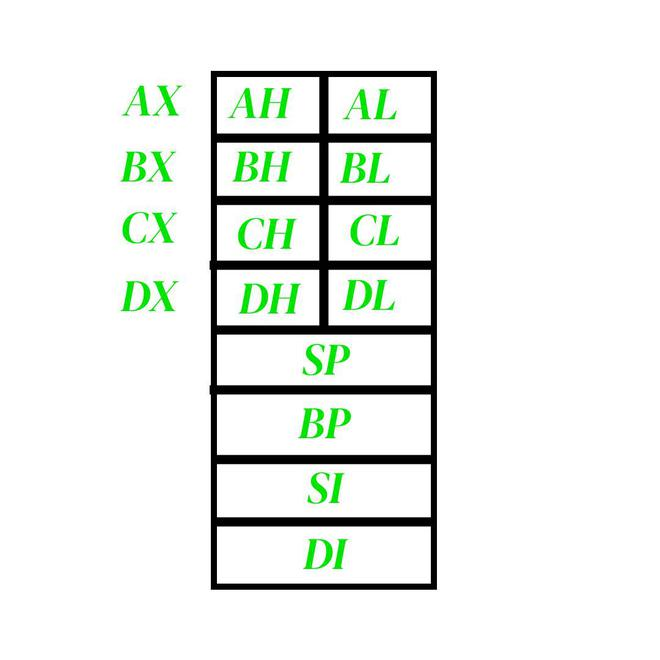Open in App
Not now

# General purpose registers in 8086 microprocessor

• Difficulty Level : Basic
• Last Updated : 24 Jun, 2022

General-purpose registers are used to store temporary data within the microprocessor. There are 8 general-purpose registers in the 8086 microprocessor.1. AX: This is the accumulator. It is of 16 bits and is divided into two 8-bit registers AH and AL to also perform 8-bit instructions. It is generally used for arithmetical and logical instructions but in 8086 microprocessor it is not mandatory to have an accumulator as the destination operand. Example:

`ADD AX, AX (AX = AX + AX)`

2. BX: This is the base register. It is of 16 bits and is divided into two 8-bit registers BH and BL to also perform 8-bit instructions. It is used to store the value of the offset. Example:

`MOV BL,  (BL = 500H)`

3. CX: This is the counter register. It is of 16 bits and is divided into two 8-bit registers CH and CL to also perform 8-bit instructions. It is used in looping and rotation. Example:

```MOV CX, 0005
LOOP```

4. DX: This is the data register. It is of 16 bits and is divided into two 8-bit registers DH and DL to also perform 8-bit instructions. It is used in the multiplication and input/output port addressing. Example:

`MUL BX (DX, AX = AX * BX)`

5. SP: This is the stack pointer. It is of 16 bits. It points to the topmost item of the stack. If the stack is empty the stack pointer will be (FFFE)H. Its offset address is relative to the stack segment.

6. BP – This is the base pointer. It is of 16 bits. It is primarily used in accessing parameters passed by the stack. Its offset address is relative to the stack segment.

7. SI – This is the source index register. It is of 16 bits. It is used in the pointer addressing of data and as a source in some string-related operations. Its offset is relative to the data segment.

8. DI – This is the destination index register. It is of 16 bits. It is used in the pointer addressing of data and as a destination in some string-related operations. Its offset is relative to the extra segment.

My Personal Notes arrow_drop_up
Related Articles